Miscellaneous

Chapter 11 Class 12 Three Dimensional Geometry
Serial order wise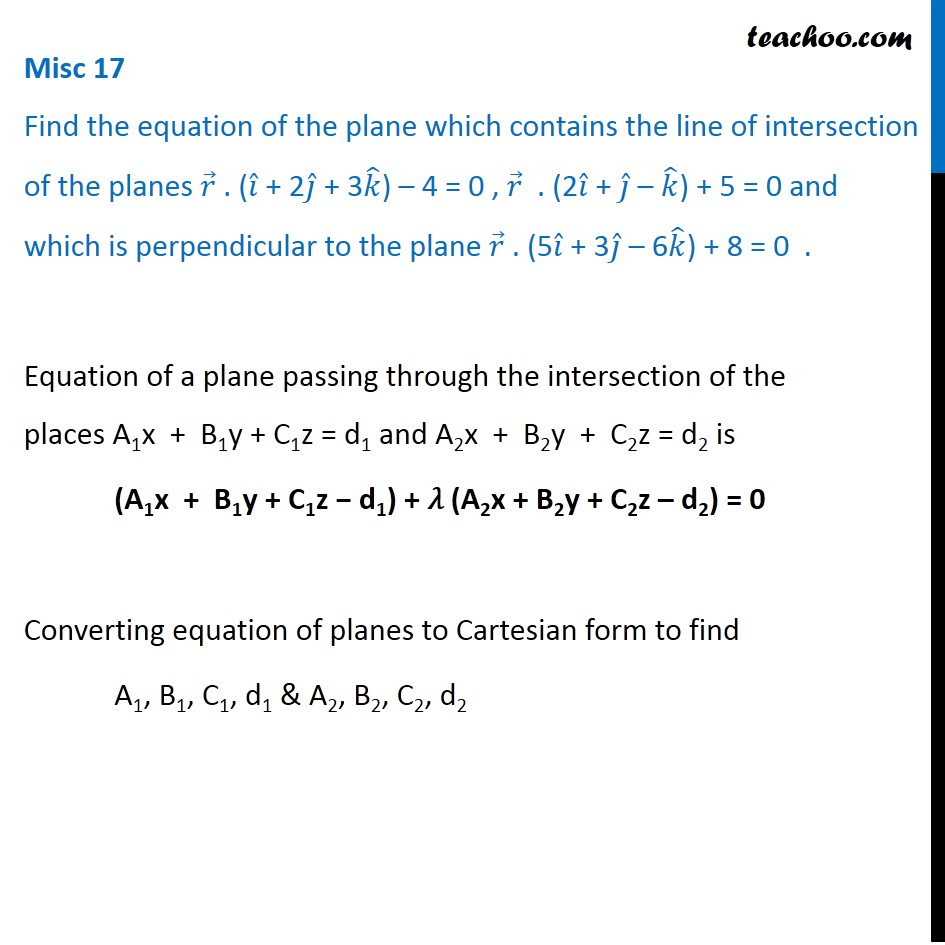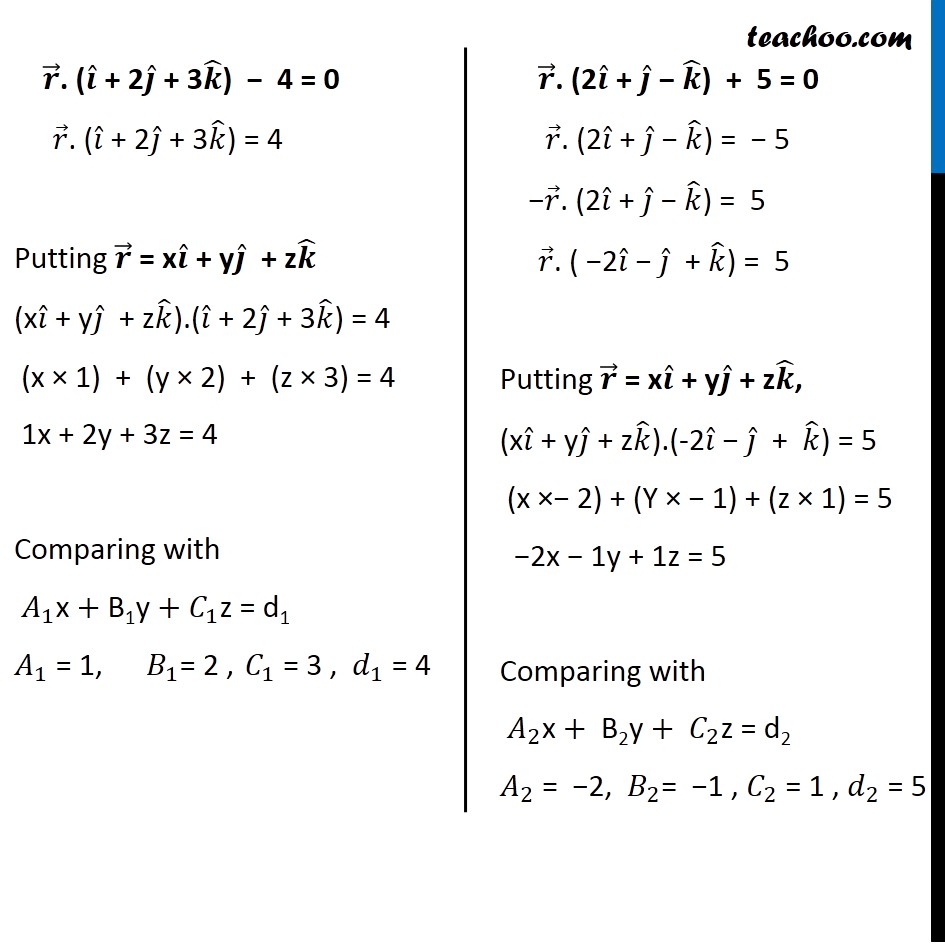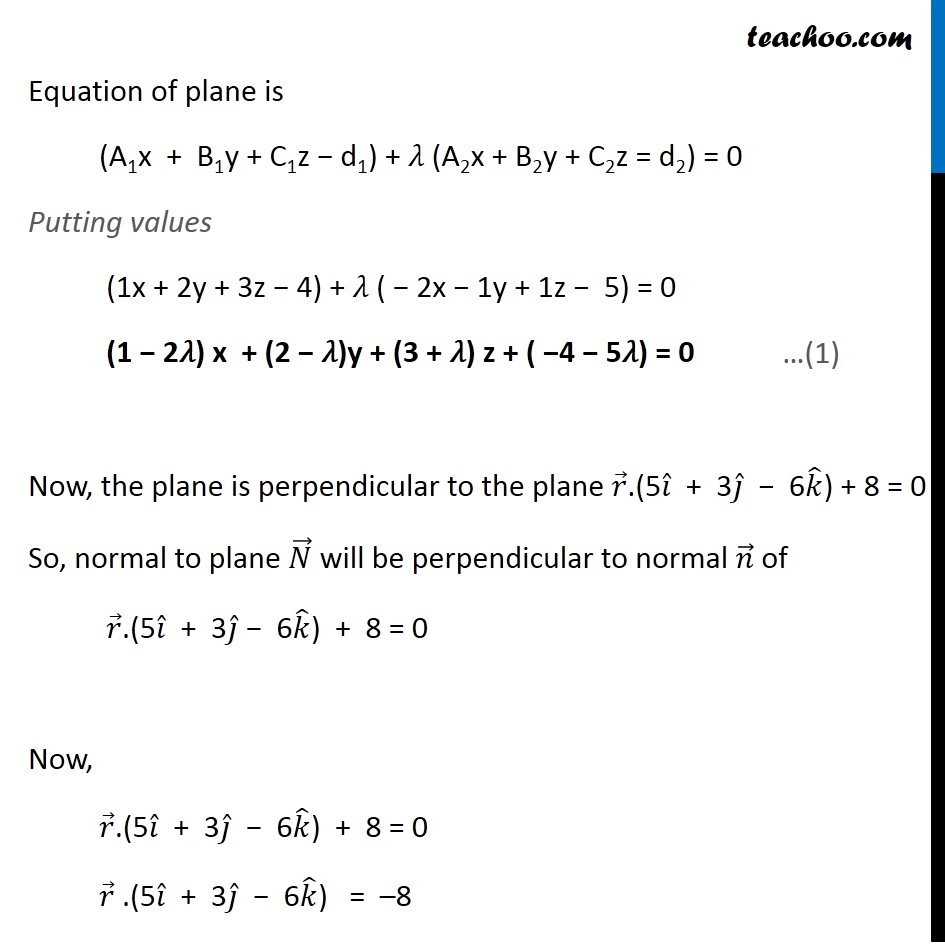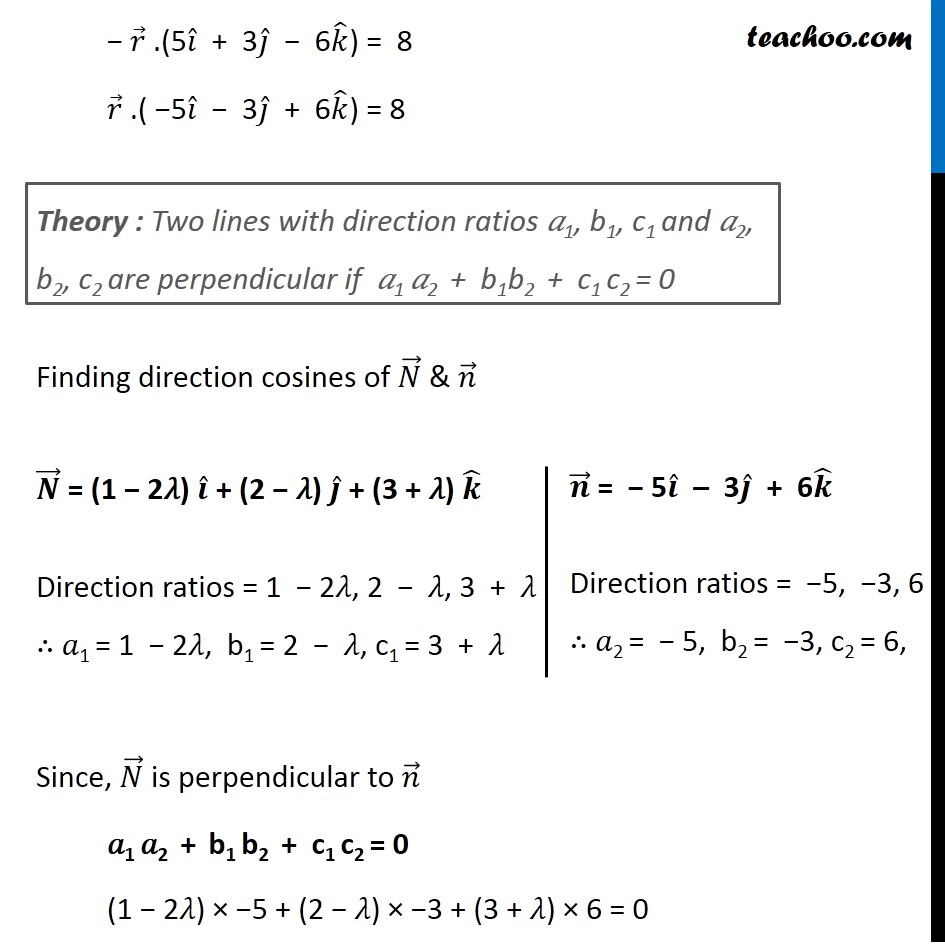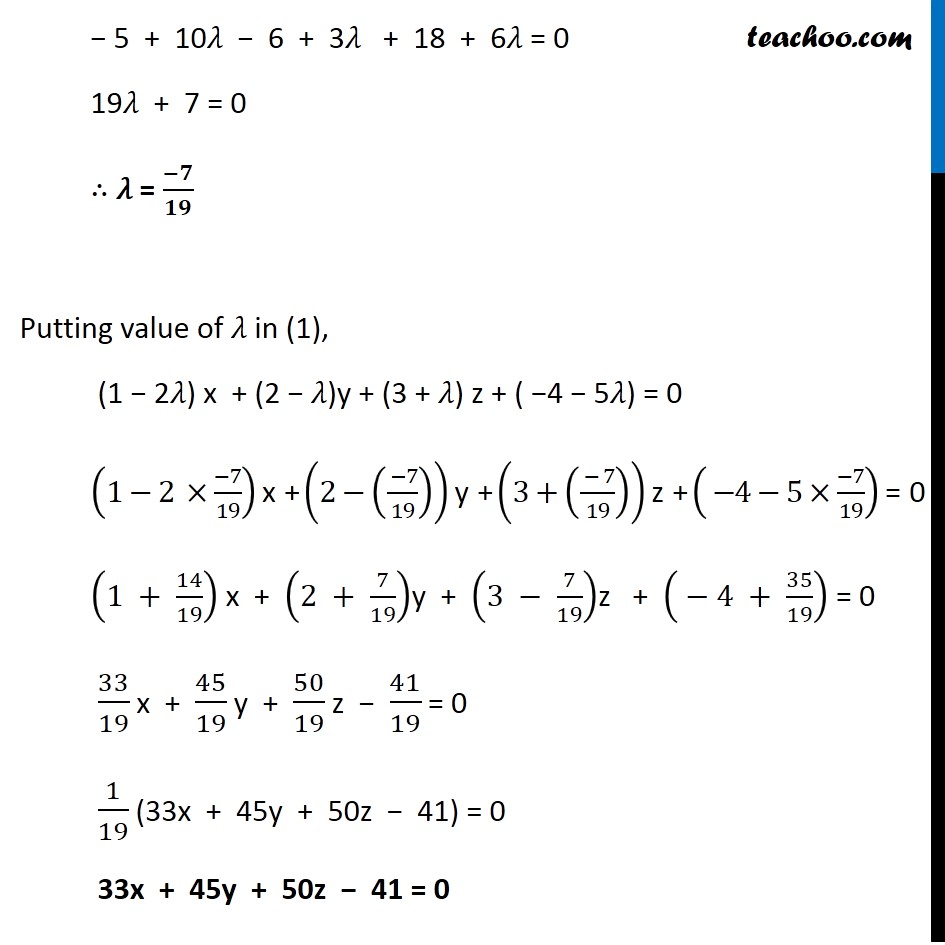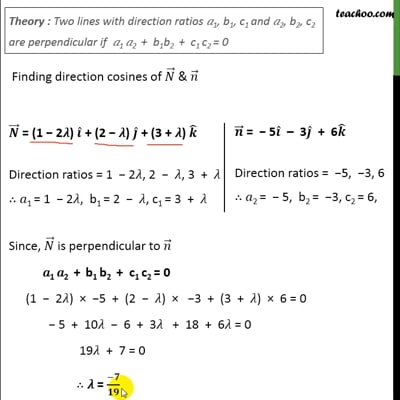This video is only available for Teachoo black users

Learn in your speed, with individual attention - Teachoo Maths 1-on-1 Class

### Transcript

Question 13 Find the equation of the plane which contains the line of intersection of the planes 𝑟 ⃗ . (𝑖 ̂ + 2𝑗 ̂ + 3𝑘 ̂) – 4 = 0 , 𝑟 ⃗ . (2𝑖 ̂ + 𝑗 ̂ – 𝑘 ̂) + 5 = 0 and which is perpendicular to the plane 𝑟 ⃗ . (5𝑖 ̂ + 3𝑗 ̂ – 6𝑘 ̂) + 8 = 0 .Equation of a plane passing through the intersection of the places A1x + B1y + C1z = d1 and A2x + B2y + C2z = d2 is (A1x + B1y + C1z − d1) + 𝜆 (A2x + B2y + C2z – d2) = 0 Converting equation of planes to Cartesian form to find A1, B1, C1, d1 & A2, B2, C2, d2 𝒓 ⃗. (𝒊 ̂ + 2𝒋 ̂ + 3𝒌 ̂) − 4 = 0 𝑟 ⃗. (𝑖 ̂ + 2𝑗 ̂ + 3𝑘 ̂) = 4 Putting 𝒓 ⃗ = x𝒊 ̂ + y𝒋 ̂ + z𝒌 ̂ (x𝑖 ̂ + y𝑗 ̂ + z𝑘 ̂).(𝑖 ̂ + 2𝑗 ̂ + 3𝑘 ̂) = 4 (x × 1) + (y × 2) + (z × 3) = 4 1x + 2y + 3z = 4 Comparing with 𝐴_1 "x"+"B1y"+𝐶_1 "z = d1" 𝐴_1 = 1, 𝐵_1= 2 , 𝐶_1 = 3 , 𝑑_1 = 4 𝒓 ⃗. (2𝒊 ̂ + 𝒋 ̂ − 𝒌 ̂) + 5 = 0 𝑟 ⃗. (2𝑖 ̂ + 𝑗 ̂ − 𝑘 ̂) = − 5 −𝑟 ⃗. (2𝑖 ̂ + 𝑗 ̂ − 𝑘 ̂) = 5 𝑟 ⃗. ( −2𝑖 ̂ − 𝑗 ̂ + 𝑘 ̂) = 5 Putting 𝒓 ⃗ = x𝒊 ̂ + y𝒋 ̂ + z𝒌 ̂, (x𝑖 ̂ + y𝑗 ̂ + z𝑘 ̂).(-2𝑖 ̂ − 𝑗 ̂ + 𝑘 ̂) = 5 (x ×− 2) + (Y × − 1) + (z × 1) = 5 −2x − 1y + 1z = 5 Comparing with 𝐴_2 "x"+ "B2y"+ 𝐶_2 "z = d2" 𝐴_2 = −2, 𝐵_2= −1 , 𝐶_2 = 1 , 𝑑_2 = 5 Equation of plane is (A1x + B1y + C1z − d1) + 𝜆 (A2x + B2y + C2z = d2) = 0 Putting values (1x + 2y + 3z − 4) + 𝜆 ( − 2x − 1y + 1z − 5) = 0 (1 − 2𝜆) x + (2 − 𝜆)y + (3 + 𝜆) z + ( −4 − 5𝜆) = 0 Now, the plane is perpendicular to the plane 𝑟 ⃗.(5𝑖 ̂ + 3𝑗 ̂ − 6𝑘 ̂) + 8 = 0 So, normal to plane 𝑁 ⃗ will be perpendicular to normal 𝑛 ⃗ of 𝑟 ⃗.(5𝑖 ̂ + 3𝑗 ̂ − 6𝑘 ̂) + 8 = 0 Now, 𝑟 ⃗.(5𝑖 ̂ + 3𝑗 ̂ − 6𝑘 ̂) + 8 = 0 𝑟 ⃗ .(5𝑖 ̂ + 3𝑗 ̂ − 6𝑘 ̂) = –8 − 𝑟 ⃗ .(5𝑖 ̂ + 3𝑗 ̂ − 6𝑘 ̂) = 8 𝑟 ⃗ .( −5𝑖 ̂ − 3𝑗 ̂ + 6𝑘 ̂) = 8 Finding direction cosines of 𝑁 ⃗ & 𝑛 ⃗ Since, 𝑁 ⃗ is perpendicular to 𝑛 ⃗ 𝑎1 𝑎2 + b1 b2 + c1 c2 = 0 (1 − 2𝜆) × −5 + (2 − 𝜆) × −3 + (3 + 𝜆) × 6 = 0 Theory : Two lines with direction ratios 𝑎1, b1, c1 and 𝑎2, b2, c2 are perpendicular if 𝑎1 𝑎2 + b1b2 + c1 c2 = 0 𝑵 ⃗ = (1 − 2𝜆) 𝒊 ̂ + (2 − 𝜆) 𝒋 ̂ + (3 + 𝜆) 𝒌 ̂ Direction ratios = 1 − 2𝜆, 2 − 𝜆, 3 + 𝜆 ∴ 𝑎1 = 1 − 2𝜆, b1 = 2 − 𝜆, c1 = 3 + 𝜆 𝒏 ⃗ = − 5𝒊 ̂ – 3𝒋 ̂ + 6𝒌 ̂ Direction ratios = −5, −3, 6 ∴ 𝑎2 = − 5, b2 = −3, c2 = 6, − 5 + 10𝜆 − 6 + 3𝜆 + 18 + 6𝜆 = 0 19𝜆 + 7 = 0 ∴ 𝜆 = (−𝟕)/𝟏𝟗 Putting value of 𝜆 in (1), (1 − 2𝜆) x + (2 − 𝜆)y + (3 + 𝜆) z + ( −4 − 5𝜆) = 0 (1−2 ×(−7)/19) x + (2−(( −7)/19)) y + (3+(( − 7)/19)) z + ( −4−5×(−7)/19) = 0 (1 + 14/19) x + (2 + 7/19)y + (3 − 7/19)z + ( − 4 + 35/19) = 0 33/19 x + 45/19 y + 50/19 z − 41/19 = 0 1/19 (33x + 45y + 50z − 41) = 0 33x + 45y + 50z − 41 = 0